Categories:

Updated:

# Distributions

Definition:Set of all possible random variables

## Types of Distributions

### Uniform Distribution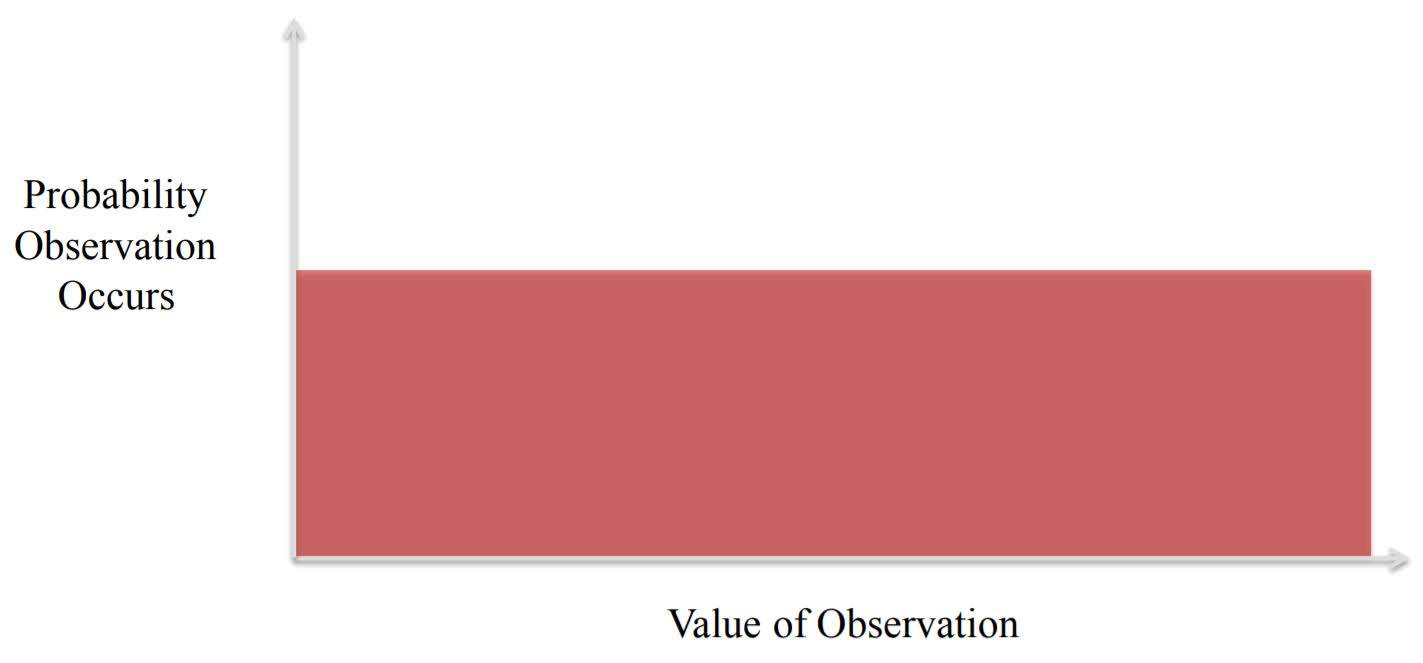### Normal (Gaussian) Distribution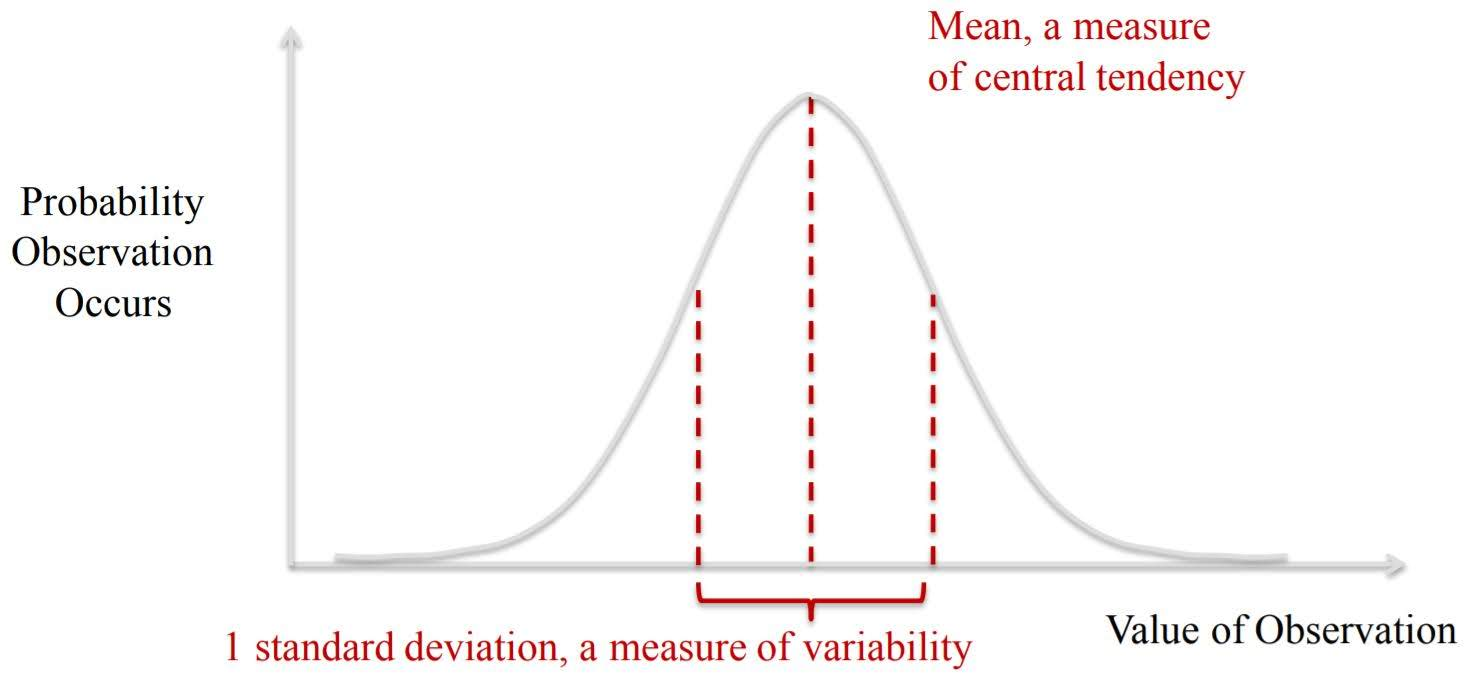Formula for standard deviation: $\sqrt{\frac{1}{N} \sum_{i=1}^N (x_i - \overline{x})^2}$

### Chi Squared Distribution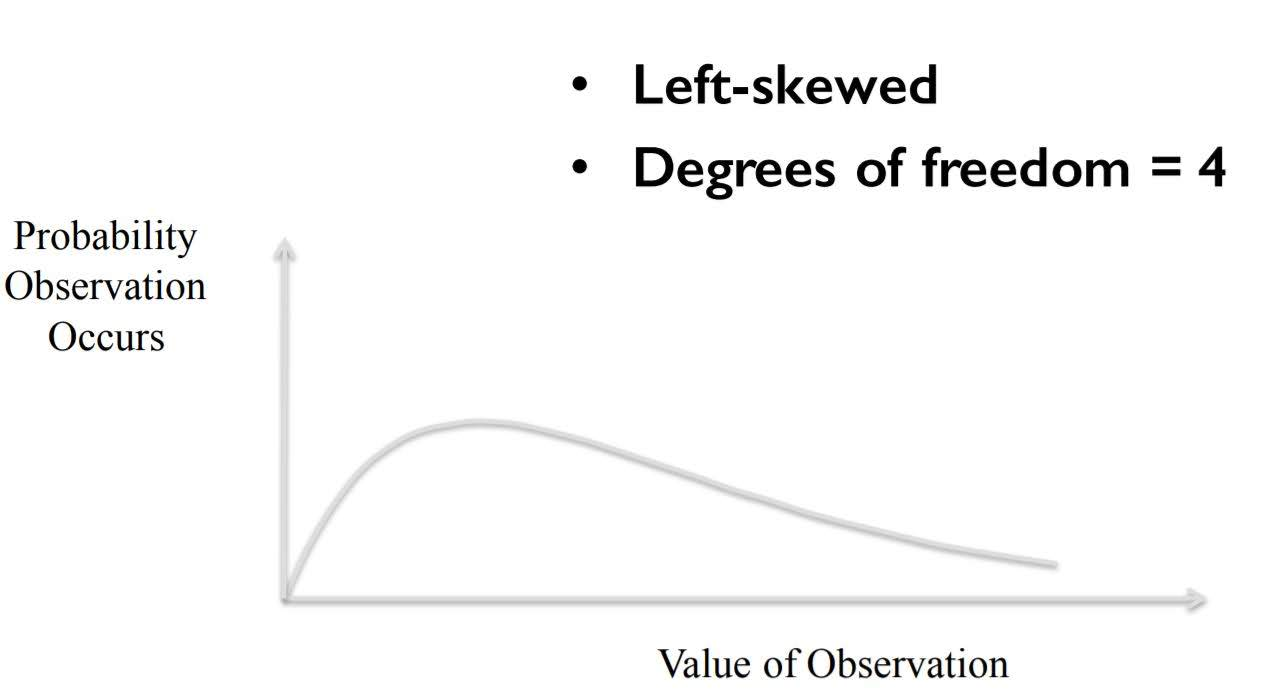Skewness & Kurtosis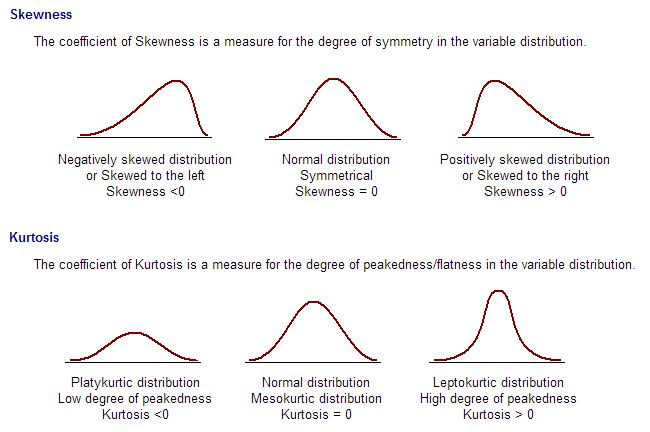Skewness is a measure of symmetry, or more precisely, the lack of symmetry. A distribution, or data set, is symmetric if it looks the same to the left and right of the center point.
When ‘Degrees of Freedom’ gets smaller, the graph gets skewed to left.

Kurtosis is a measure of whether the data are heavy-tailed or light-tailed relative to a normal distribution. That is, data sets with high kurtosis tend to have heavy tails, or outliers. Data sets with low kurtosis tend to have light tails, or lack of outliers. A uniform distribution would be the extreme case.

### Bimodal distributions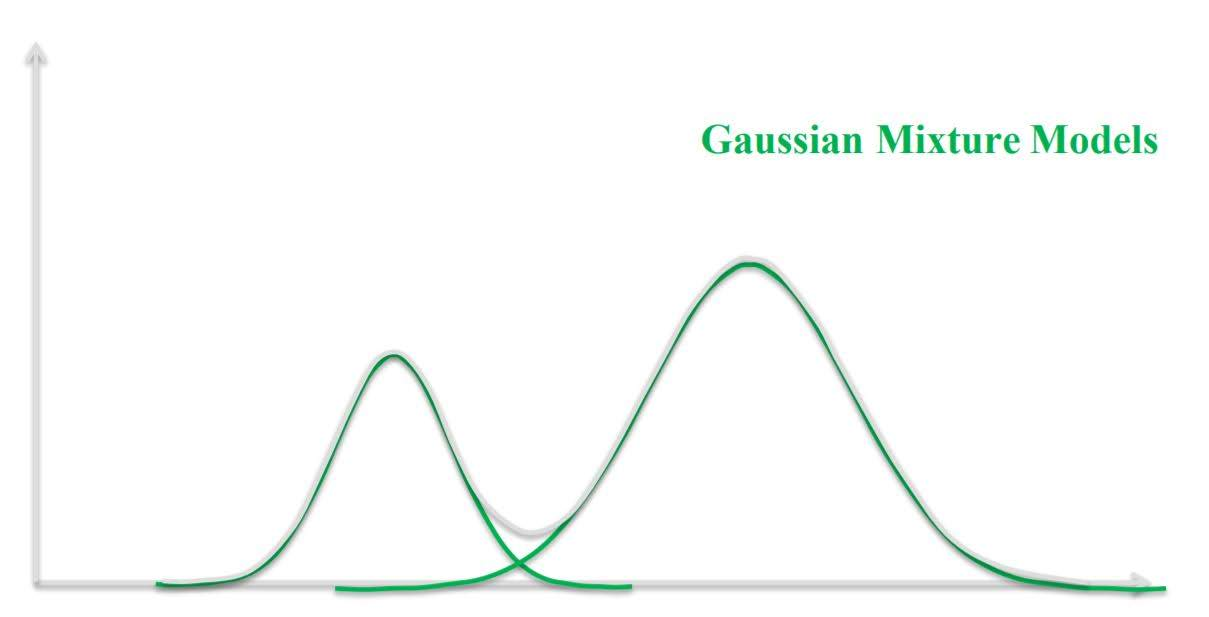# Distributions in Pandas

import pandas as pd
import numpy as np

np.random.binomial(1, 0.5)

1

# Flip 1000 coins and check number of heads. Do this 10 times.
np.random.binomial(1000, 0.5, 10)

array([502, 511, 516, 494, 527, 512, 529, 521, 495, 487])

# sample a number from uniform distribution between 0 and 1
np.random.uniform(0, 1)

0.2828033347414428

#sample a number from normald distribution of mean=0, std=1
np.random.normal(loc=0, scale=1)

-1.3560071953448096

distribution = np.random.normal(loc=0, scale=1, size=1000)
distribution.std()

0.9831044712693359

import scipy.stats as stats
stats.kurtosis(distribution)

0.025384635432275093

stats.skew(distribution)

0.055923877445990366

chi_squared_df2 = np.random.chisquare(2, size=10000)
stats.skew(chi_squared_df2)

2.0018163864318907

chi_squared_df5 = np.random.chisquare(5, size=10000)
stats.skew(chi_squared_df5)

1.3161735810606243

%matplotlib inline
import matplotlib
import matplotlib.pyplot as plt
import seaborn as sns
sns.set()

plt.figure(figsize=(14,8))
plt.hist([chi_squared_df2,chi_squared_df5], bins=100, label=['2 degrees of freedom','5 degrees of freedom'], histtype='stepfilled', alpha=0.6)
plt.legend()

<matplotlib.legend.Legend at 0x17d0be8e7f0>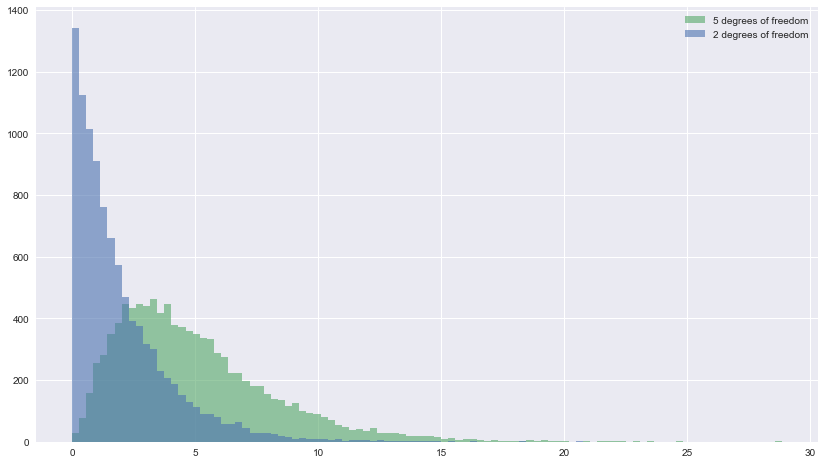# Hypothesis Testing

P-value, or critical value $\alpha$ of hypothesis testing of a model shows probability that the correlation happend just on chance.
Typically in social sciences, we accept our hypothesis if p-value is less than 0.1, 0.05, or 0.01.

We will use ttest_ind.
We can use this test, if we observe two independent samples from the same or different population, e.g. exam scores of boys and girls or of two ethnic groups. The test measures whether the average (expected) value differs significantly across samples. If we observe a large p-value, for example larger than 0.05 or 0.1, then we cannot reject the null hypothesis of identical average scores. If the p-value is smaller than the threshold, e.g. 1%, 5% or 10%, then we reject the null hypothesis of equal averages.

df = pd.read_csv('grades.csv')

df.head()

0 B73F2C11-70F0-E37D-8B10-1D20AFED50B1 92.733946 2015-11-02 06:55:34.282000000 83.030552 2015-11-09 02:22:58.938000000 67.164441 2015-11-12 08:58:33.998000000 53.011553 2015-11-16 01:21:24.663000000 47.710398 2015-11-20 13:24:59.692000000 38.168318 2015-11-22 18:31:15.934000000
1 98A0FAE0-A19A-13D2-4BB5-CFBFD94031D1 86.790821 2015-11-29 14:57:44.429000000 86.290821 2015-12-06 17:41:18.449000000 69.772657 2015-12-10 08:54:55.904000000 55.098125 2015-12-13 17:32:30.941000000 49.588313 2015-12-19 23:26:39.285000000 44.629482 2015-12-21 17:07:24.275000000
2 D0F62040-CEB0-904C-F563-2F8620916C4E 85.512541 2016-01-09 05:36:02.389000000 85.512541 2016-01-09 06:39:44.416000000 68.410033 2016-01-15 20:22:45.882000000 54.728026 2016-01-11 12:41:50.749000000 49.255224 2016-01-11 17:31:12.489000000 44.329701 2016-01-17 16:24:42.765000000
3 FFDF2B2C-F514-EF7F-6538-A6A53518E9DC 86.030665 2016-04-30 06:50:39.801000000 68.824532 2016-04-30 17:20:38.727000000 61.942079 2016-05-12 07:47:16.326000000 49.553663 2016-05-07 16:09:20.485000000 49.553663 2016-05-24 12:51:18.016000000 44.598297 2016-05-26 08:09:12.058000000
4 5ECBEEB6-F1CE-80AE-3164-E45E99473FB4 64.813800 2015-12-13 17:06:10.750000000 51.491040 2015-12-14 12:25:12.056000000 41.932832 2015-12-29 14:25:22.594000000 36.929549 2015-12-28 01:29:55.901000000 33.236594 2015-12-29 14:46:06.628000000 33.236594 2016-01-05 01:06:59.546000000
len(df)

2315

early = df[df['assignment1_submission'] <= '2015-12-31']
late = df[df['assignment1_submission'] > '2015-12-31']

early.mean()

assignment1_grade    74.972741
dtype: float64

late.mean()

assignment1_grade    74.017429
dtype: float64

from scipy import stats

stats.ttest_ind(early['assignment1_grade'], late['assignment1_grade'])

Ttest_indResult(statistic=1.400549944897566, pvalue=0.16148283016060577)

stats.ttest_ind(early['assignment2_grade'], late['assignment2_grade'])

Ttest_indResult(statistic=1.3239868220912567, pvalue=0.18563824610067967)

stats.ttest_ind(early['assignment3_grade'], late['assignment3_grade'])

Ttest_indResult(statistic=1.7116160037010733, pvalue=0.08710151634155668)


According to ttest_ind, mean value of assignment3 grade between early students and late students might be correlated.

Categories: